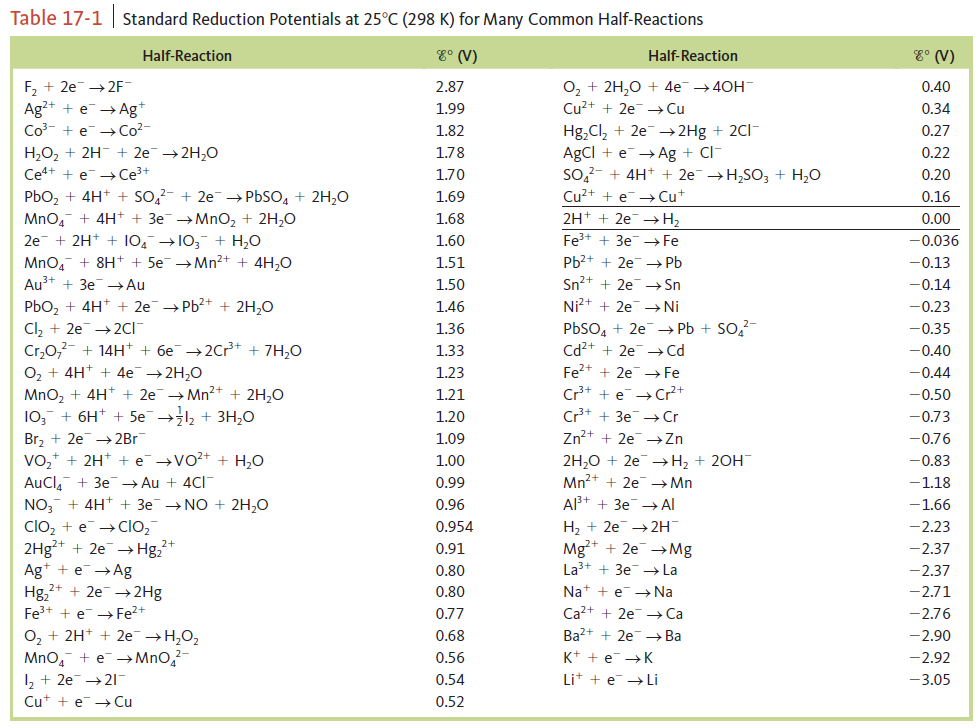# Problem: Consider only the species (at standard conditions)Br -, Br2, H+, H2, La3+, Ca, Cdin answering the following questions. Give reasons for your answers. (Use data from Table 17‑1.)a. Which is the strongest oxidizing agent?

###### FREE Expert Solution
84% (127 ratings)
###### Problem Details

Consider only the species (at standard conditions)

Br -, Br2, H+, H2, La3+, Ca, Cd

in answering the following questions. Give reasons for your answers. (Use data from Table 17‑1.)

a. Which is the strongest oxidizing agent?Frequently Asked Questions

What scientific concept do you need to know in order to solve this problem?

Our tutors have indicated that to solve this problem you will need to apply the Redox Reaction concept. You can view video lessons to learn Redox Reaction. Or if you need more Redox Reaction practice, you can also practice Redox Reaction practice problems.

What textbook is this problem found in?

Our data indicates that this problem or a close variation was asked in Chemistry: An Atoms First Approach - Zumdahl Atoms 1st 2nd Edition. You can also practice Chemistry: An Atoms First Approach - Zumdahl Atoms 1st 2nd Edition practice problems.